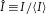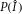## Main

Most text books on condensed matter explain that the electronic states in disordered conductors are extended plane or Bloch waves with finite lifetimes. This gives rise to ‘ohmic resistance’, proportional to the length of the sample. In the picture presented by Anderson1, ‘large’ disorder makes electronic states localized in space. This offers a mechanism to explain the widely observed metal–insulator transitions17. Scaling theory proposes a single parameter, the Thouless conductance g, to describe the anomalous length dependence of the resistance of a sample2. The localized regime is characterized by the so-called Thouless criterion g<1. Although these ideas were first proposed for electron localization, in the early 1980s interest in classical-wave localization was raised18,19, with the promise of avoiding the difficulties caused by interactions in electronic systems. At the same time, the absence of bound states for classical waves makes localization more challenging to achieve in practice, with absorption as an extra concern.

Here, we demonstrate Anderson localization of ultrasound in a three-dimensional (3D) medium. Our samples are single-component random networks made by brazing aluminium beads together, see Fig. 1a. With ultrasound, we probe the vibrational excitations of the network in the intermediate frequency regime (0.2–3 MHz), where the wavelength is comparable to the bead and pore sizes. We use pulsed techniques to measure the amplitude transmission coefficient, shown in Fig. 1b. The transmission spectrum exhibits a succession of bandgaps and pass bands, due to the overlapping resonances of the aluminium beads20. Our study focuses on frequencies just below the first bandgap at 0.5 MHz, and at higher frequencies (1.6–3 MHz), where it was possible to extract the phase and amplitude of the coherent pulse for longitudinal waves crossing the sample. Hence, we were able to measure the longitudinal phase vp and group vg velocities, as well as the scattering mean free path ℓ (refs 21, 22). Note that because absorption is negligible (see below), the attenuation of the coherent pulse gives ℓ directly. Around 0.20 MHz, vp=1.75 km s−1, vg=2.1 km s−1 and ℓ=2.2 mm, whereas around 2.4 MHz, vp=5.0 km s−1, vg=5.2 km s−1 and ℓ=0.6 mm. This leads to a product of wave vector k and scattering mean free path kℓ=1.6 at 0.20 MHz and kℓ=1.8 at 2.4 MHz. The values of kℓ for shear waves, likely to dominate, are not known but are probably roughly equal23. The small values of kℓ indicate that our samples are strongly scattering. For 3D disorder, localization is expected when k1 (ref. 24). Because the exact critical value is not known, the ultrasonic waves at both frequencies are candidates to be Anderson localized.

In previous reports on Anderson localization with classical waves, absorption has been a major obstacle to reaching unambiguous conclusions7,8,10,11,25. The following experiment is capable of probing Anderson localization without being blurred by absorption. We measure the spatially and time-resolved transmitted intensity through our sample. Using a quasi-point source that is about a wavelength wide and a subwavelength-diameter detector that scans the sample at various transverse positions ρ in the near field (ρ=0 opposite to the source), the average transmitted intensity I(ρ,t) was determined26. From these measurements, we determine the ratio I(ρ,t)/I(0,t), probing the dynamic spreading of the intensity in the transverse direction. Any possible absorption factor exp(−t/τa) cancels in this ratio. For each ρ, a transverse width wρ(t) of I(ρ,t) can be defined by setting I(ρ,t)/I(0,t)≡exp[−ρ2/wρ2(t)]. If the wave propagation is diffuse, the spatial intensity profile is Gaussian and wρ2(t)=4D t is independent of ρ. We have observed this normal diffuse behaviour in less strongly scattering samples, and hence have been able to demonstrate a way of measuring the diffusion coefficient without the usual complications due to boundary conditions and absorption26.

For frequencies between 1.6 and 3 MHz, we observe markedly different behaviour, shown in Fig. 2 for two representative samples at 2.4 MHz. Instead of increasing linearly with propagation time, wρ2(t) is seen to saturate. This saturation is reminiscent of transverse localization, previously predicted in 3D systems with 2D disorder27 and observed in 2D disordered photonic crystals12. In our samples, the disorder is clearly 3D because the scattering mean free path is much smaller than the sample thicknesses (10<L/ℓ<40). It is not at all clear a priori that transverse localization can occur with 3D disorder. Scaling theory2 predicts a diffusivity D(L) dependent on the sample size L, so that wρ2(t)=4D(L)t still rises linearly with time. The saturation of wρ(t) could possibly be explained by a diffusivity D(t) decaying with time9,10,11. This would still lead to a Gaussian transverse intensity profile and hence to wρ2 constant with ρ. However, this is not what we observe.

To describe the dynamics of the anomalous transverse confinement of the multiply scattered waves, we apply a novel version of the self-consistent theory of localization. The new element consists of incorporating boundary conditions self-consistently28,29. This theory provides a position-dependent, dynamic diffusivity kernel D(r,tt′). Near the mobility edge, the position dependence of D affects all aspects of wave transport considerably. The self-consistent theory requires as input the value for kℓ, the localization length ξ, the diffusion constant DB free from macroscopic interferences and the internal reflection coefficient R. In the model, we replace the incident focused beam by a point source at depth ℓB*. This is the transport mean free path associated with diffusion in the absence of macroscopic interferences, which ought to be negligible just after the beam comes in. Figure 2 compares the observed dynamics of transverse width to this theory. Excellent agreement with the data is seen for all ρ with a single set of parameters for each sample (solid curves), yielding, in particular, ξ≈15 mm and ξ≈7 mm for the thinner and thicker samples respectively. We attribute the smaller value of ξ for the thicker sample to stronger scattering due to small differences in the microstructure. This is consistent with the strong sensitivity of ξ to small changes in disorder that is expected near the localization transition. As ρ increases, the curves wρ2(t) move upwards, meaning that the observed transverse profile I(ρ,t) is not Gaussian. Figure 2 shows that this behaviour is well captured by the theory, in which the position dependence of D is a crucial element. Any homogeneous absorption would not affect the results in Fig. 2. We believe that this combination of theory and experiment provides strong evidence for Anderson localization of ultrasound near 2.4 MHz in our samples and enables us to estimate the mobility edge (kℓ)c, which we find to be approximately 1% above the measured kℓ=1.8.

To find extra support for our conclusions, we have measured the time-dependent transmission using an extended quasi-plane-wave source. Near 0.2 MHz, the average transmitted intensity I(t) was found to decay exponentially at long times (Fig. 3a), with the entire time dependence of I(t) being well described by diffusion theory26. We conclude that multiply scattered ultrasound at 0.2 MHz propagates in a normal, diffuse way. In contrast, for ultrasound propagating near 2.4 MHz, the time dependence of I(t) shows a quite different behaviour (Fig. 3b), with a non-exponential tail at long times. Similar behaviour has been reported before and is often explained by a time-dependent reduction of the effective diffusion coefficient D(t) (refs 9, 10, 11). The solid curve in Fig. 3b is a fit to the self-consistent theory, and gives an excellent description of the experiment at all propagation times. The good agreement between theory and experiment supports our previous conclusion that ultrasound at 2.4 MHz is localized. From the fits in Figs 2 and 3b and the relation DB=vEB*/3, we find transport velocities 3–5 times larger than the phase velocity of longitudinal waves. Further theoretical work is needed to understand these—apparently large—values for vE in the localized regime.

We also address the statistical approach to Anderson localization7. The normalized transmitted intensitywas measured for a large number of individual speckle spots in the near field, and for a broad incident beam. The intensity distributionsfor both frequencies are shown in Fig. 4. We have compared our data to the theory of ref. 30, with the Thouless conductance g as the only free parameter in the fit. The agreement is excellent for g=0.80±0.08 at 2.4 MHz and g=11.4±0.8 at 0.2 MHz. The strong deviation from Rayleigh statistics with g<1 observed at 2.4 MHz is interpreted as a signature of localization7. The remarkable agreement with theory, derived for the intensity in the far field, and for g1, reveals a universality of the statistics of localized waves.

The normalized intensityat a particular frequency was determined from the square of the magnitude of the Fourier transform of the transmitted field in each near-field speckle, normalized by the average intensity in the speckle pattern. The results were then binned to determine. To improve the statistics, the results were averaged over up to 100 frequencies within a 5% bandwidth where the statistics were similar—the same bandwidth as for the dynamic measurements of I(ρ,t) and I(t). In the localized regime, where fluctuations are largest, the statistical accuracy of the measured distribution was further improved by averaging over different samples, which were found to exhibit the same statistics. This enabled the error bars in Fig. 4d to be determined from the standard error in the mean.